# ePSproc $$\beta_{L,M}$$ calculations demo

10/09/19

Source notebook on Github.

## Basic IO

:

import sys
import os
import time
import numpy as np

# For module testing, include path to module here
modPath = r'D:\code\github\ePSproc'
sys.path.append(modPath)
import epsproc as ep

* pyevtk not found, VTK export not available.

:

# Load data from modPath\data
dataPath = os.path.join(modPath, 'data')

# Scan data dir

*** ePSproc readMatEle(): scanning files for DumpIdy segments (matrix elements)

*** Scanning dir
D:\code\github\ePSproc\data
Found 2 .out file(s)

*** Reading ePS output file:  D:\code\github\ePSproc\data\n2_3sg_0.1-50.1eV_A2.inp.out
Expecting 2 symmetries.
Expecting 102 dumpIdy segments.
Found 102 dumpIdy segments (sets of matrix elements).

Processing segments to Xarrays...
Processed 102 sets of matrix elements (0 blank)

*** Reading ePS output file:  D:\code\github\ePSproc\data\no2_demo_ePS.out
Expecting 3 symmetries.
Expecting 3 dumpIdy segments.
Found 3 dumpIdy segments (sets of matrix elements).

Processing segments to Xarrays...
Processed 3 sets of matrix elements (0 blank)


## Formalism

The $$\beta_{L,M}$$ parameters are defined as:

$\begin{eqnarray} \beta_{L,-M}^{\mu_{i},\mu_{f}} & = & \sum_{l,m,\mu}\sum_{l',m',\mu'}(-1)^{M}(-1)^{m}(-1)^{(\mu'-\mu_{0})}\left(\frac{(2l+1)(2l'+1)(2L+1)}{4\pi}\right)^{1/2}\left(\begin{array}{ccc} l & l' & L\\ 0 & 0 & 0 \end{array}\right)\left(\begin{array}{ccc} l & l' & L\\ -m & m' & -M \end{array}\right)\nonumber \\ & \times & \sum_{P,R',R}(2P+1)(-1)^{(R'-R)}\left(\begin{array}{ccc} 1 & 1 & P\\ \mu & -\mu' & R' \end{array}\right)\left(\begin{array}{ccc} 1 & 1 & P\\ \mu_{0} & -\mu_{0} & R \end{array}\right)D_{-R',-R}^{P}(R_{\hat{n}})I_{l,m,\mu}^{p_{i}\mu_{i},p_{f}\mu_{f}}(E)I_{l',m',\mu'}^{p_{i}\mu_{i},p_{f}\mu_{f}*}(E) \end{eqnarray}$

Calculations use ep.mfblm(), which will calculate all values at each energy point for the supplied dataset. This may take a while in some cases due to multiple nested sums - this code will be parallelised in future.

## $$N_2$$ mutli-E

Calculate $$\beta_{LM}$$ as function of energy.

:

daIn = dataSet.copy()

# BLMXeN2 = ep.mfblm(daIn[:, 1:4], selDims = {'Type':'L'}, thres = 1e-4)       # Subselected on Eke
start = time.time()
BLMXeN2 = ep.mfblm(daIn, selDims = {'Type':'L'}, thres = 1e-4)   # Run for all Eke
end = time.time()

Calculating MFBLMs for 81 pairs... Eke = 0.1 eV, eAngs = ([0, 0, 0])
Calculating MFBLMs for 81 pairs... Eke = 1.1 eV, eAngs = ([0, 0, 0])
Calculating MFBLMs for 81 pairs... Eke = 2.1 eV, eAngs = ([0, 0, 0])
Calculating MFBLMs for 81 pairs... Eke = 3.1 eV, eAngs = ([0, 0, 0])
Calculating MFBLMs for 81 pairs... Eke = 4.1 eV, eAngs = ([0, 0, 0])
Calculating MFBLMs for 81 pairs... Eke = 5.1 eV, eAngs = ([0, 0, 0])
Calculating MFBLMs for 81 pairs... Eke = 6.1 eV, eAngs = ([0, 0, 0])
Calculating MFBLMs for 81 pairs... Eke = 7.1 eV, eAngs = ([0, 0, 0])
Calculating MFBLMs for 81 pairs... Eke = 8.1 eV, eAngs = ([0, 0, 0])
Calculating MFBLMs for 81 pairs... Eke = 9.1 eV, eAngs = ([0, 0, 0])
Calculating MFBLMs for 81 pairs... Eke = 10.1 eV, eAngs = ([0, 0, 0])
Calculating MFBLMs for 81 pairs... Eke = 11.1 eV, eAngs = ([0, 0, 0])
Calculating MFBLMs for 81 pairs... Eke = 12.1 eV, eAngs = ([0, 0, 0])
Calculating MFBLMs for 81 pairs... Eke = 13.1 eV, eAngs = ([0, 0, 0])
Calculating MFBLMs for 81 pairs... Eke = 14.1 eV, eAngs = ([0, 0, 0])
Calculating MFBLMs for 81 pairs... Eke = 15.1 eV, eAngs = ([0, 0, 0])
Calculating MFBLMs for 81 pairs... Eke = 16.1 eV, eAngs = ([0, 0, 0])
Calculating MFBLMs for 81 pairs... Eke = 17.1 eV, eAngs = ([0, 0, 0])
Calculating MFBLMs for 81 pairs... Eke = 18.1 eV, eAngs = ([0, 0, 0])
Calculating MFBLMs for 81 pairs... Eke = 19.1 eV, eAngs = ([0, 0, 0])
Calculating MFBLMs for 81 pairs... Eke = 20.1 eV, eAngs = ([0, 0, 0])
Calculating MFBLMs for 81 pairs... Eke = 21.1 eV, eAngs = ([0, 0, 0])
Calculating MFBLMs for 81 pairs... Eke = 22.1 eV, eAngs = ([0, 0, 0])
Calculating MFBLMs for 81 pairs... Eke = 23.1 eV, eAngs = ([0, 0, 0])
Calculating MFBLMs for 81 pairs... Eke = 24.1 eV, eAngs = ([0, 0, 0])
Calculating MFBLMs for 81 pairs... Eke = 25.1 eV, eAngs = ([0, 0, 0])
Calculating MFBLMs for 81 pairs... Eke = 26.1 eV, eAngs = ([0, 0, 0])
Calculating MFBLMs for 81 pairs... Eke = 27.1 eV, eAngs = ([0, 0, 0])
Calculating MFBLMs for 81 pairs... Eke = 28.1 eV, eAngs = ([0, 0, 0])
Calculating MFBLMs for 81 pairs... Eke = 29.1 eV, eAngs = ([0, 0, 0])
Calculating MFBLMs for 81 pairs... Eke = 30.1 eV, eAngs = ([0, 0, 0])
Calculating MFBLMs for 81 pairs... Eke = 31.1 eV, eAngs = ([0, 0, 0])
Calculating MFBLMs for 81 pairs... Eke = 32.1 eV, eAngs = ([0, 0, 0])
Calculating MFBLMs for 81 pairs... Eke = 33.1 eV, eAngs = ([0, 0, 0])
Calculating MFBLMs for 81 pairs... Eke = 34.1 eV, eAngs = ([0, 0, 0])
Calculating MFBLMs for 81 pairs... Eke = 35.1 eV, eAngs = ([0, 0, 0])
Calculating MFBLMs for 81 pairs... Eke = 36.1 eV, eAngs = ([0, 0, 0])
Calculating MFBLMs for 81 pairs... Eke = 37.1 eV, eAngs = ([0, 0, 0])
Calculating MFBLMs for 81 pairs... Eke = 38.1 eV, eAngs = ([0, 0, 0])
Calculating MFBLMs for 81 pairs... Eke = 39.1 eV, eAngs = ([0, 0, 0])
Calculating MFBLMs for 81 pairs... Eke = 40.1 eV, eAngs = ([0, 0, 0])
Calculating MFBLMs for 81 pairs... Eke = 41.1 eV, eAngs = ([0, 0, 0])
Calculating MFBLMs for 81 pairs... Eke = 42.1 eV, eAngs = ([0, 0, 0])
Calculating MFBLMs for 81 pairs... Eke = 43.1 eV, eAngs = ([0, 0, 0])
Calculating MFBLMs for 81 pairs... Eke = 44.1 eV, eAngs = ([0, 0, 0])
Calculating MFBLMs for 81 pairs... Eke = 45.1 eV, eAngs = ([0, 0, 0])
Calculating MFBLMs for 81 pairs... Eke = 46.1 eV, eAngs = ([0, 0, 0])
Calculating MFBLMs for 81 pairs... Eke = 47.1 eV, eAngs = ([0, 0, 0])
Calculating MFBLMs for 81 pairs... Eke = 48.1 eV, eAngs = ([0, 0, 0])
Calculating MFBLMs for 81 pairs... Eke = 49.1 eV, eAngs = ([0, 0, 0])
Calculating MFBLMs for 81 pairs... Eke = 50.1 eV, eAngs = ([0, 0, 0])

:

BLMXeN2

:

<xarray.DataArray (Euler: 1, Eke: 51, BLM: 121)>
array([[[2.301322 +0.j, 0.       +0.j, ...,      nan+nanj,      nan+nanj],
[2.382333 +0.j, 0.       +0.j, ...,      nan+nanj,      nan+nanj],
...,
[0.092948 +0.j, 0.       +0.j, ..., 0.       +0.j, 0.       +0.j],
[0.095428 +0.j, 0.       +0.j, ..., 0.       +0.j, 0.       +0.j]]])
Coordinates:
* Euler    (Euler) MultiIndex
- P        (Euler) int64 0
- T        (Euler) int64 0
- C        (Euler) int64 0
* BLM      (BLM) MultiIndex
- l        (BLM) int64 0 1 1 1 2 2 2 2 2 3 3 ... 10 10 10 10 10 10 10 10 10 10
- m        (BLM) int64 0 -1 0 1 -2 -1 0 1 2 -3 -2 ... 0 1 2 3 4 5 6 7 8 9 10
* Eke      (Eke) float64 0.1 1.1 2.1 3.1 4.1 5.1 ... 46.1 47.1 48.1 49.1 50.1
Attributes:
Lmax:      11
Targ:      SG
QNs:       ['m', 'l', 'mu', 'ip', 'it', 'Value']
dataType:  BLM
file:      n2_3sg_0.1-50.1eV_A2.inp.out
fileBase:  D:\code\github\ePSproc\data
thres:     0.0001
sumDims:   ('l', 'm', 'mu', 'Cont', 'Targ', 'Total', 'it')
selDims:   [('Type', 'L')]

:

# Plot using Xarray functionality with thresholding
BLMXeN2.where(np.abs(BLMXeN2) > 1e-4, drop = True).real.squeeze().plot.line(x='Eke')

:

[<matplotlib.lines.Line2D at 0x1fd4a9a24a8>,
<matplotlib.lines.Line2D at 0x1fd4a9a2fd0>,
<matplotlib.lines.Line2D at 0x1fd4a9a27b8>,
<matplotlib.lines.Line2D at 0x1fd4b84dc18>,
<matplotlib.lines.Line2D at 0x1fd4b84d940>,
<matplotlib.lines.Line2D at 0x1fd4b84d6a0>]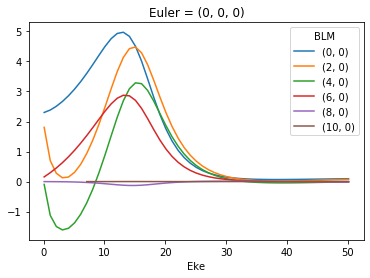:

# Plot values normalised by B00
# This seems to work... probably a more elegant solution here, since this assumes dimension order.
normBLM = BLMXeN2/BLMXeN2[:,:,0]
normBLM.where(np.abs(normBLM) > 1e-4, drop = True).real.squeeze().plot.line(x='Eke')

:

[<matplotlib.lines.Line2D at 0x1fd4adb56a0>,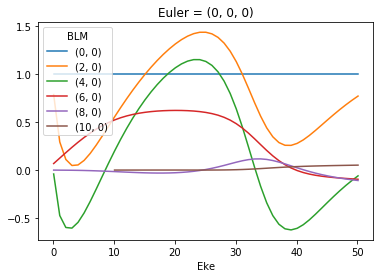### MFPADs: calculate & plot from $$\beta_{LM}$$

:

# Calculate & plot MFPADs from BLMs
#     # Calculate YLMs
#     YLMX = ep.sphCalc(BLMXin.l.max(), res=50)
#     YLMX = YLMX.rename({'LM':'BLM'})    # Switch naming for multiplication & plotting


:

# Plot single E with matplotlib
ep.sphSumPlotX(MFPAD_N2.sel({'Eke':1.1}).squeeze(), pType = 'r', backend = 'mpl')

Plotting with mpl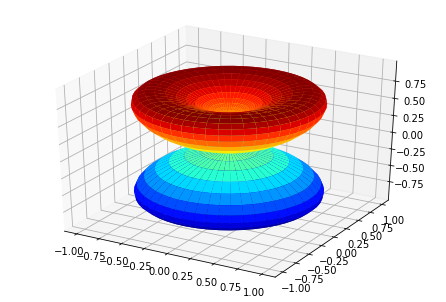:

[<Figure size 432x288 with 1 Axes>]

:

# Plot MFPAD surfaces vs E
print('N2 test data, MFPADs vs E')


N2 test data, MFPADs vs E

:

<xarray.plot.facetgrid.FacetGrid at 0x1fd4b91f048>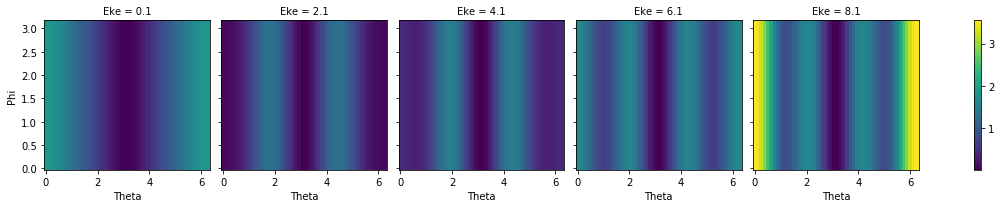:

# Plot multiple E with matplotlib
ep.sphSumPlotX(MFPAD_N2.isel(Eke=slice(0,50,10)), pType = 'r', backend = 'mpl')

Plotting with mpl
Data dims: ('Euler', 'Eke', 'Theta', 'Phi'), subplots on Eke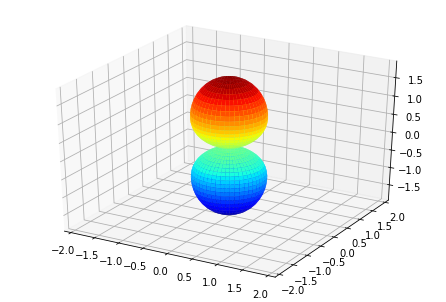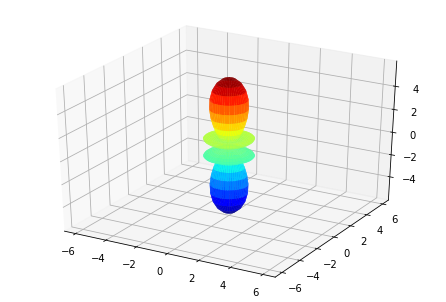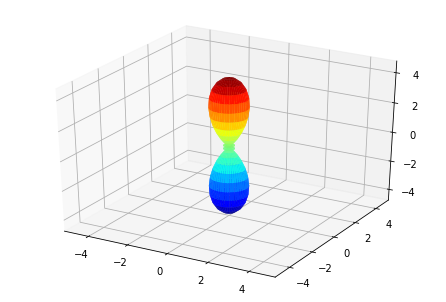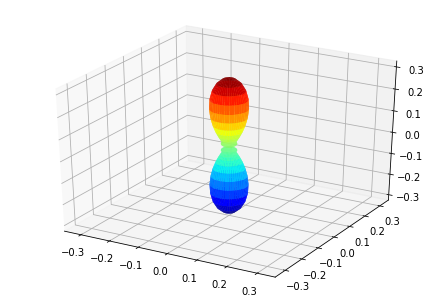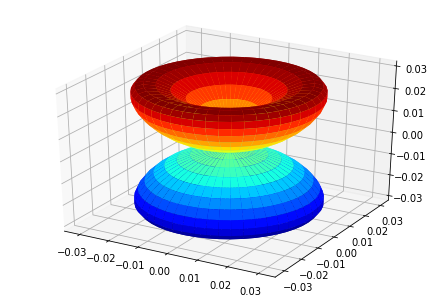:

[<Figure size 432x288 with 1 Axes>,
<Figure size 432x288 with 1 Axes>,
<Figure size 432x288 with 1 Axes>,
<Figure size 432x288 with 1 Axes>,
<Figure size 432x288 with 1 Axes>]

:

# Plot multiple E with plotly (work in progress!)
# Broken since adding Euler angs...?
_ = ep.sphSumPlotX(MFPAD_N2.squeeze().isel(Eke=slice(0,50,10)), pType = 'r', backend = 'pl')

Plotting with pl